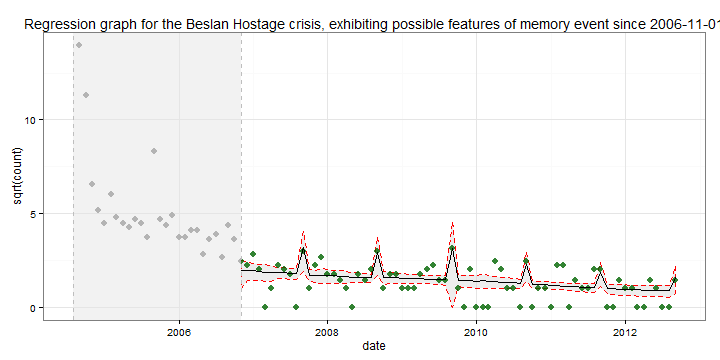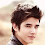## document.write(ssyby);

### Better modelling and visualisation of newspaper count data

<!-- Styles for R syntax highlighterIn this post I outline how count data may be modelled using a negative binomial distribution in order to more accurately present trends in time series count data than using linear methods. I also show how to use ANOVA to identify the point at which one model gains explanatory power, and how confidence intervals may be calculated and plotted around the predicted values. The resulting illustration gives a robust visualisation of how the Beslan Hostage crisis has taken on features of a memory event
Recently I wrote up a piece about quantifying memory and news, and proposed that two distinct linear models might be the way to go about it. However, the problem with linear models is they by their nature don't take into account the ways in which trends may be non-linear.They also lead to nonsense predictions, such as negative values.
Generally, then, linear models should be avoided when mapping count data. What are the alternatives? Typically, a Poisson distribution would be ideal way to capture the probability of a clustering of observations being non-random. A feature of the Poisson distribution is that it assumes the sample mean equals the sample variance; this is very frequently violated when dealing with news data, as a story will have a small number of large values, followed by a large number of small values, resulting in a low mean and a high variance. Instead a negative binomial distribution may be used, which takes a value theta specifying the degree to which the variance and mean are unequal. Estimates provided by a negative binomial model are the same as Poisson estimates, but probability values tend to be more conservative.
One strength of R is its ability to model so called generalised linear models. The negative binomial distribution comes from the package MASS; theta may be calculated using glm.nb:
``````library(MASS)
fmla <- as.formula("count~date+e_news+a1+a2+elections")
theta <- glm.nb(fmla, data = mdb)\$theta
results <- glm(fmla, data = mdb, family = negative.binomial(theta))
``````
The above results may be considered in ANOVA to identify which variables contribute significantly to the model.
``````anova(results)
``````
``````Analysis of Deviance Table

Response: count

Terms added sequentially (first to last)

Df Deviance Resid. Df Resid. Dev
NULL                         96        994
date       1      731        95        263
e_news     1       69        94        194
a1         1       52        93        142
a2         1        1        92        141
elections  1       12        91        129
``````
Details about the coding of the variables, and the logic behind models contrasting stories as news or memory events may be found here.
From the ANOVA results I can identify which group of variables are contributing the most substantially to describing the data distribution: memory variables, or news variables. As I am interested in distributions where memory effects are apparent, and these develop only over time, I loop through the data deleting the first month's values, until such a time as there is no data left, or the memory variables have greater explanatory power than the news estimator:
``````mdb2 <- mdb  #copy the data
n <- 0
news <- 0
memory <- 0
while (n == 0) {
aov3 <- glm(fmla, data = mdb2, family = negative.binomial(glm.nb(fmla, data = mdb2)\$theta))
anova(aov3)
t <- data.frame(t(anova(aov3)))
news <- sum(t[, grep("date|news", colnames(t))])
memory <- sum(t[, grep("a1|a1", colnames(t))])
if (news > memory) {
n <- 0
mdb2 <- mdb2[mdb2\$date > min(mdb2\$date), ]
} else (n <- 1)
}
``````
From the negative binomial model predictions and confidence intervals for the period identified as of potential memory significance may be created. The 95% confidence interval either side of the predicted value is calculated by multiplying the standard error by 1.96. I want to plot the whole data, but only predicted values for the period identified as significant, so next I removed predictions for the data up until the period with memory potential. Finally I created a data frame containing the interval for which no estimates were calculated (this will be used to blur out data in ggplot).
``````estimate <- (predict.glm(aov3, newdata = mdb, type = "response", se.fit = T))
mdb\$estimate <- estimate\$fit
mdb\$se <- estimate\$se.fit
mdb\$estimate[mdb\$date < min(mdb2\$date)] <- NA
mdb\$se[mdb\$date < min(mdb2\$date)] <- NA
mdb\$date <- as.Date(mdb\$date)
mdb\$upper <- mdb\$estimate + (1.96 * mdb\$se)
mdb\$lower <- mdb\$estimate - (1.96 * mdb\$se)
mdb\$lower[mdb\$lower < 0] <- 0
rect <- data.frame(min(mdb\$date) - months(1), min(as.Date(mdb2\$date)))
colnames(rect) <- c("one", "two")
``````
In the plot below I visualise the square root of articles about the Beslan hostage tragedy in the Russian press. The square root is chosen to prevent the high initial interest from obscuring the trend that emerged over time. To create the plot I
• add a ribbon representing the confidence interval
• plot the observed values
• add a dotted line representing the fitted values
• edit the formatting and add a title
• add a shaded rectangle over the area I wish to ignore:
``````ggplot(mdb, aes(date, sqrt(count), ymax = sqrt(upper), ymin = sqrt(lower)),
environment = environment()) + geom_ribbon(colour = "red", fill = "light grey",
alpha = 0.4, linetype = 2) + geom_point(colour = "dark green", size = 3,
alpha = 0.8) + geom_line(aes(date, sqrt(estimate))) + theme_bw() + ggtitle(paste0("Regression graph for the Beslan Hostage crisis, exhibiting possible features of memory event since ",
as.Date(min(mdb2\$date)))) + geom_rect(aes(xmin = rect\$one, xmax = rect\$two,
ymin = -Inf, ymax = +Inf), fill = "light grey", colour = "grey", linetype = 2,
alpha = 0.015)
````````````anova(aov3)
``````
``````Analysis of Deviance Table

Response: count

Terms added sequentially (first to last)

Df Deviance Resid. Df Resid. Dev
NULL                         70      135.3
date       1    19.18        69      116.1
e_news     1     0.73        68      115.4
a1         1    24.54        67       90.9
a2         1     0.65        66       90.2
elections  1     0.29        65       89.9
``````
Notice in the above table how the anniversaries variables exceed the explanatory power of the news and date variables. This indicates that by the end of 2006 Beslan was increasingly featuring as a memory event and less as a news story. Also notice how the remaining deviance is quite large - this model apparently fits the data less well than the model for the entire data (it explained 85% of the deviance), but this is due to the original estimate being biased by the accurate prediction of a few outliers.
-->

1.I'am glad to read the whole content of this blog and am very excited,Thank you for sharing good topic.

ดูหนังออนไลน์

2.KARNA RASA HATI YANG GEMBIRA BERKAT BANTUAN AKI SOLEH
MAKANYA SENGAJA NAMA BELIAU SAYA CANTUNKAN DI INTERNET !!!

assalamualaikum wr, wb, saya IBU PUSPITA WATI saya Mengucapkan banyak2
atas nomor togelnya yang kemarin AKI berikan "4D"
alhamdulillah ternyata itu benar2 tembus AKI
dan berkat bantuan AKI SOLEH saya bisa melunasi semua hutan2…
orang tua saya yang ada di BANK BRI dan bukan hanya itu AKI alhamdulillah,
sekarang saya sudah bisa bermodal sedikit untuk mencukupi kebutuhan keluarga saya sehari2.
Itu semua berkat bantuan AKI SOLEH sekali lagi makasih banyak ya, AKI
yang ingin merubah nasib
seperti saya...?
SILAHKAN GABUNG SAMA AKI SOLEH No; { 082-313-336-747 }

Sebelum Gabung Sama AKI Baca Duluh Kata2 Yang Dibawah Ini
Apakah anda termasuk dalam kategori di bawah ini...!!
1: Di kejar2 tagihan hutang..
2: Selaluh kalah dalam bermain togel
3: Barang berharga sudah
terjual buat judi togel..
4: Sudah kemana2 tapi tidak
menghasilkan, solusi yang tepat..!
5: Sudah banyak dukun ditempati minta angka ritual blom dapat juga,
satu jalan menyelesaikan masalah anda..
Dijamin anda akan berhasil
silahkan buktikan sendiri
Atau Chat/Tlpn di WhatsApp (WA)
No WA Aki : 082313336747

TERIMA KASIH YANG PUNYA
ROOM ATAS TUMPANGANYA SALAM KOMPAK SELALU
"KLIK DISINI BOCORAN TOGEL SGP HK SDY DAN DLL"

3.Amazing Article ! I would like to thank you for the efforts you had made for writing this awesome article.
Thanks for sharing such a nice info.I hope you will share more information like this. please keep on sharing!

internship in chennai
internship in chennai for cse
internship for mba in chennai
internship in chennai for hr
internship in chennai for mba
companies for internship in chennai
internship in chennai for ece
paid internship in chennai
internship in chennai for biotechnology
internship in chennai for b.com students

4.5.6.I always appreciated your work, your creation is definitely unique. Great job
rasmussen student portal

7.Hvala na ispravci. ažuriramo turske serije sa prevodom. gledajte online turske serije online sa prevodom
https://turskeserije.org/

8.cryptoanime is honest website and there reviews recommendation is best

9.This comment has been removed by the author.